# Metal Catalog

Metal catalog, conductors

Use the `metal` and `MetalCatalog` functions to select and customize different metals used in printed circuit boards (PCB). Explore different conductor materials and model the conductivity and thickness of the metal to optimize the PCB design and reduce resistive losses.

Metal Catalog

 PEC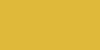σ = Infd = 0 Copper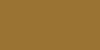σ = 59.6e06 S/md = 3.556e-05 m Aluminum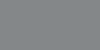σ = 37.7e06 S/md = 7.62e-04 m Gold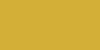σ = 41.1e06 S/md = 2e-07 m Silver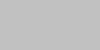σ = 63e06 S/md = 2e-07 m Custom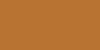σ = User definedd = User defined Zincσ = 16.9e06 S/md = 1.016e-04 m Tungsten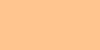σ = 17.9e06 S/md = 2e-07 m Lead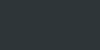σ = 4.55e06 S/md = 2e-07 m Iron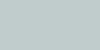σ = 10e06 S/md = 2e-07 m Steel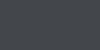σ = 6.99e06 S/md = 0.68e-03 m Brass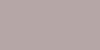σ = 15.9e06 S/md = 0.68e-03 m

## Functions

 `metal` Conductor material `MetalCatalog` Catalog of metals